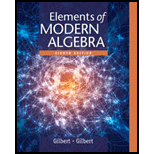# Let A = R − { 0 } , the set of all nonzero real numbers, and consider the following relations on A × A . Decide in each case whether R is an equivalence relation, and justify your answers. ( a , b ) R ( c , d ) if and only if a d = b c . ( a , b ) R ( c , d ) if and only if a b = c d . ( a , b ) R ( c , d ) if and only if a 2 + b 2 = c 2 + d 2 . ( a , b ) R ( c , d ) if and only if a − b = c − d .### Elements Of Modern Algebra

8th Edition
Gilbert + 2 others
Publisher: Cengage Learning,
ISBN: 9781285463230### Elements Of Modern Algebra

8th Edition
Gilbert + 2 others
Publisher: Cengage Learning,
ISBN: 9781285463230

#### Solutions

Chapter
Section
Chapter 1.7, Problem 15E
Textbook Problem

## Expert Solution

### Want to see the full answer?

Check out a sample textbook solution.See solution

### Want to see this answer and more?

Experts are waiting 24/7 to provide step-by-step solutions in as fast as 30 minutes!*

See Solution

*Response times vary by subject and question complexity. Median response time is 34 minutes and may be longer for new subjects.